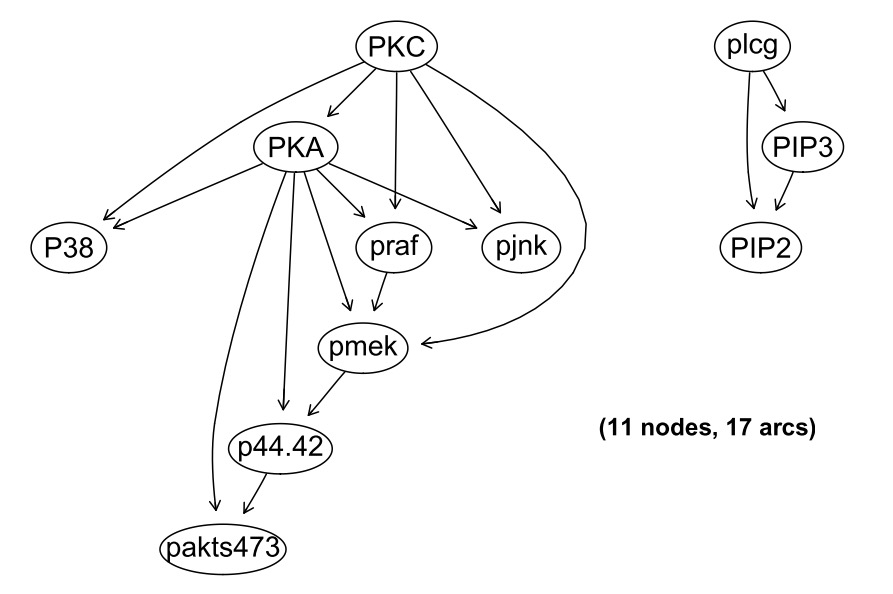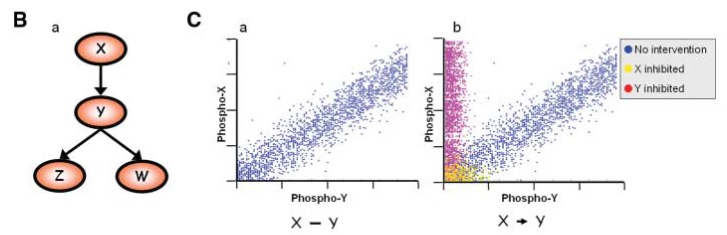library(ggplot2)
library(reshape2)
library(Ckmeans.1d.dp)
library(corrplot)
library(bnlearn)
library(igraph)
library(Rgraphviz)

# Part 1: constraint vs. score based approaches

We have generated a dataset with 3 random variables A, B, C; using constraint-based and score based approaches, we try to infer the structure of the networks

data = readRDS(gzcon(url('https://www.dropbox.com/s/s9hqxf423i2hkmx/data2.rds?dl=1')))
head(data)

## Contraint based approaches

Using the function ci.test with the test="mi" method, test:

• the independence of each pair of variables
• the independence of each pair of variables, given the third
ci.test('A','C',data=data,test='mi')
ci.test('A','C','B',data=data,test='mi')
ci.test('B','C',data=data,test='mi')
ci.test('B','C','A',data=data,test='mi')
ci.test('B','A',data=data,test='mi')
ci.test('B','A','C',data=data,test='mi')

Can you determine the possible architectures of the BN?

## Score based approaches

Using constraint-based approaches, we cannot fully resolve the structure of the network

net = iamb(data)
graphviz.plot(net)

We can now try to orient the edges manually, and compute the score (e.g. AIC score) of the oriented network

net1 = set.arc(net,'A' ,'B')
net1 = set.arc(net1,'C' ,'B')
graphviz.plot(net1)

We can determine the parameters (i.e. the conditional probabilities) of the network:

net1.fit = bn.fit(net1,data=data)
net1.fit
## computing the BIC score of the network
bnlearn::score(net1,data)

Test the other possible structures of the network; can you find the most likely one(s)?

# Part 2 : T-cell Pathway reconstruction (Sachs et al., 2005)

Topics:

• discretization strategies
• bootstrapping and consensus networks
• using intervention data

This dataset consists of single-cell cytometry data on 11 proteins in a primary T-cell signaling network, using normal condition (“observational”) or perturbed conditions (“interventional”). http://www.sciencemag.org/cgi/doi/10.1126/science.1105809Literature validated network

## exploring the observational data

sachs = read.table('https://www.dropbox.com/s/fcq3cqxheklr2it/sachs.data.txt?dl=1',header=TRUE)

tmp = melt(sachs)
p = ggplot(tmp,aes(x=value, fill=variable, color=variable)) +
geom_density(alpha=0.30,size=0.7) + theme_bw() +
facet_wrap(~variable, scales = "free") + labs(x="log2(value)")+
theme(legend.title = element_blank(),
panel.grid.major = element_blank(),
panel.grid.minor = element_blank())

rm(tmp)
p

## Discretizing the data

We discretize the data, since it does not follow the assumption of normal distribution.

## discretize the data
dsachs = discretize(sachs,method='hartemink',breaks = 3, ibreaks = 60, idisc = 'quantile')

tmp=melt(dsachs,id.vars=c())
p = ggplot(tmp,aes(x=value, fill=variable, color=variable)) +
geom_bar() + theme_bw() +
facet_wrap(~variable, scales = "free") + labs(x="Categories")+
theme(legend.title = element_blank(),
panel.grid.major = element_blank(),
panel.grid.minor = element_blank()
)

p
head(dsachs)

## Learning the BN from discretized data

boot = boot.strength(data=dsachs, R=500,
algorithm='hc',
algorithm.args = list(score='bde')
)

dim(boot)

## now threshold the edge strength and the direction evidence

boot.filt = boot[(boot$strength > 0.85 & boot$direction > 0.5),]

dim(boot.filt)
boot.filt

## Averaging the network

We have learned 500 networks starting from different initial random networks; we can now average these networks.

avg.boot = averaged.network(boot, threshold = 0.8)
net = avg.boot$arcs net = graph_from_edgelist(net,directed=T) library(Rgraphviz) graphviz.plot(avg.boot) ## Use interventional data for Sachs So far the network was built using observational data, i.e. data collected in normal conditions. The dataset however contained additional interventional data, resulting from perturbation of specific nodes in the network. This data can either be used for validation (do the perturbation have the predicted effect from the initial network ?) or can be used to strengthen the evidence on the learned edges.Effect of interventions on the network isachs = read.table('https://www.dropbox.com/s/xegmoa1e04opc0t/sachs.interventional.txt?dl=1', header = TRUE, colClasses = "factor") head(isachs) Here, the last column indicates on which variable (i.e. column) the intervention has been performed. INT = sapply(1:11, function(x) { which(isachs$INT == x) })

isachs2 = isachs[, 1:11]
nodes = names(isachs2)
names(INT) = nodes

## we start from a set of 200 random graphs
start = random.graph(nodes = nodes,
method = "melancon",
num = 200,
burn.in = 10^5,
every = 100)

netlist = lapply(start, function(net) {
tabu(isachs2, score = "mbde", exp = INT, iss = 1, start = net, tabu = 50) }
)

arcs = custom.strength(netlist, nodes = nodes, cpdag = FALSE)

bn.mbde = averaged.network(arcs, threshold = 0.85)

net = bn.mbde$arcs net = graph_from_edgelist(net,directed=T) graphviz.plot(bn.mbde) # Part 3: building an epigenetic networks from CLL data Topics: • discretization strategies • blacklisting edges • inference Here, we want to build a chromatin network where nodes are epigenetic components, and the observations are the state of these epigenetic modifications at the promoters of genes. ## Load pre-compiled epigenetic data matrix for CLL dat = read.table('https://www.dropbox.com/s/npb177z5ceod4fw/CEMT_26-nonCGI-allDataContinuous.txt?dl=1', header = TRUE, stringsAsFactors = FALSE, sep="\t") head(dat) dim(dat) dat[,3:ncol(dat)] = round(dat[,3:ncol(dat)]/dat$Input,3)
dat = dat[,-ncol(dat)]

corVals = round(cor(dat, method="spearman"),2)

## Visualize how these epigenetic features are correlated

corrplot.mixed(corVals, tl.col = "black", tl.cex=0.8)

## Visualize how these correlated features cluster together

corrplot(corVals, order = "hclust", hclust.method = "ward.D2", addrect = 2, tl.col = "black");rm(corVals)

## Look at the distribution of the data

summary(dat)

log2dat = log2(dat+0.1)
tmp = melt(log2dat)
p = ggplot(tmp,aes(x=value, fill=variable, color=variable)) +
geom_density(alpha=0.30,size=0.7) + theme_bw() +
facet_wrap(~variable, scales = "free") + labs(x="log2(value)")+
theme(legend.title = element_blank(),
panel.grid.major = element_blank(),
panel.grid.minor = element_blank())
rm(tmp)
p

## Perform discretization using Ckmeans.1d.dp

For details regarding Ckmeans.1d.dp, see https://cran.r-project.org/web/packages/Ckmeans.1d.dp/vignettes/Ckmeans.1d.dp.html Lets check the discrete states for H3K4me3 as an example

res = Ckmeans.1d.dp(log2dat$H3K4me3) # Number of clusters predicted max(res$cluster)
# Lets look at the predictions
plot(log2dat$H3K4me3, col= res$cluster, cex=0.5, pch=20, xlab="Genes", ylab= "H3K4me3 signal")
#abline(h=res$centers, lwd=2,lty=2,col="blue") rm(res) # Compute optimal states for all states = apply(log2dat, 2, function(y){max(Ckmeans.1d.dp(x=y, k=c(1,9))$cluster)})
# For methylation lots of states are predicted, from the distribution plots
# its clear that these are mostly intermediate states
res = Ckmeans.1d.dp(log2dat$CPGfrac, k=states) plot(log2dat$CPGfrac, col= res$cluster, cex=0.5, pch=20, xlab="Genes", ylab= "CPGfrac") # so lets approximate by taking 3 states res = Ckmeans.1d.dp(log2dat$CPGfrac, k=3)
plot(log2dat$CPGfrac, col= res$cluster, cex=0.5, pch=20, xlab="Genes", ylab= "CPGfrac")
#abline(h=res$centers, lwd=2,lty=2,col="blue") # We accept all the predicted states from Ckmeans.1d.dp except for methylation # Final max discrete states are states = 3 states # Creating the discretized version of the data disDat = matrix(ncol=ncol(log2dat), nrow=nrow(log2dat)) colnames(disDat) = colnames(log2dat) rownames(disDat) = rownames(log2dat) disDat = as.data.frame(disDat) for( i in 1: length(states)) { tmp = suppressWarnings(Ckmeans.1d.dp(log2dat[,i], k=c(1,states[i]))) disDat[,i] = factor(tmp$cluster)
rm(tmp)
}
rm(i, states)

# Final view of the discretized data
summary(disDat)

# Plot the discretized data
tmp=melt(disDat,id.vars=c())
p = ggplot(tmp,aes(x=value, fill=variable, color=variable)) +
geom_bar() + theme_bw() +
facet_wrap(~variable, scales = "free") + labs(x="Categories")+
theme(legend.title = element_blank(),
panel.grid.major = element_blank(),
panel.grid.minor = element_blank()
)

p

## Learn bayesian network from the discretized data using bnlearn

We use a set of blacklist edges: no edge should go out of the expression node

# Black list .. no interaction should originate from gene expression
bl=data.frame(from="RPKM",to=colnames(disDat))
bl=bl[-which(bl$to == "RPKM"),] # Learn bayesian network via bootstrapping strength = boot.strength(disDat, algorithm="tabu", algorithm.args=list(score="aic", tabu=10, blacklist=bl), R=1000) # takes about 2mins rm(bl) # Select only those interactions meeting our filteration criteria selarcs = strength[strength$direction > 0.5 ,]
selarcs = selarcs[order(selarcs$strength,decreasing=TRUE),] selarcs # Get an averaged network dag.average.filt = averaged.network(selarcs, threshold = 0.90) knitr::kable(dag.average.filt$arcs, caption = "Interactions meeting our threshold for strength and direction")
# Compute correlations for the selected interactions
net = dag.average.filt$arcs corVals = apply(net,1, function(x){ a = log2dat[,x] b = log2dat[,x] c = round(cor(a,b,method="spearman"),2) return(c) }) # Coloring the correlation values col = rep("forestgreen",nrow(net)) col[which(corVals < 0)] = "firebrick" # Setting up the network in igraph net = graph_from_edgelist(net,directed=T) E(net)$weight = corVals
V(net)$label.color="black" rm(corVals) ## Plotting the bayesian network plot(net, edge.color=col, vertex.shape="circle", vertex.size = 40,layout=l, edge.width=E(net)$weight*3.5+3)
box();rm(col,l,pos.grp,neg.grp,left.grp,right.grp,mid.grp)

## Inference : making predictions using the network

# Fitting the learnt network to the data to obtain
# conditional probability tables
fitted = bn.fit(dag.average.filt, disDat)

# Predict Expression level by setting DNAme to high/low
x.high = as.numeric(table(cpdist(fitted,nodes=c('RPKM'),CPGfrac=='3')))

x.low = as.numeric(table(cpdist(fitted,nodes=c('RPKM'),CPGfrac=='1')))

pred = data.frame(prop=c(x.high/sum(x.high),x.low/sum(x.low)),
condition=c(rep('DNAme high',length(x.high)),rep('DNAme low',length(x.high))),
Expression=c(1:length(x.high),1:length(x.low))
)

ggplot(pred,aes(x=Expression,fill=condition,y=prop)) + geom_bar(stat='identity',position=position_dodge())
# Predict Expression level by setting H3K4me1 to high/low
x.high = as.numeric(table(cpdist(fitted,nodes=c('RPKM'),H3K4me1=='3')))
x.low = as.numeric(table(cpdist(fitted,nodes=c('RPKM'),H3K4me1=='1')))

pred = data.frame(prop=c(x.high/sum(x.high),x.low/sum(x.low)),
condition=c(rep('K4me1 high',length(x.high)),rep('K4me1 low',length(x.high))),
Expression=c(1:length(x.high),1:length(x.low))
)

ggplot(pred,aes(x=Expression,fill=condition,y=prop)) + geom_bar(stat='identity',position=position_dodge())